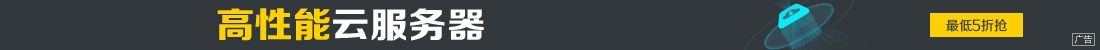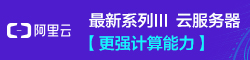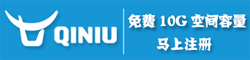# 在Oracle数据库中同时更新两张表的简单方法

••```update ZZ_TEST1 t1 set t1."text" = (
select T2."text1" from ZZ_TEST2 t2 where T2."pid"=t1."id"
)
WHERE EXISTS
(
SELECT 1 FROM ZZ_TEST2 t2 where T2."pid"=t1."id"
)
```

```update ZZ_TEST1 t1 set t1."text" = (
select T2."text1" from ZZ_TEST2 t2 where T2."pid"=t1."id"
)
where t1."id" in (select "pid" from ZZ_TEST2 )

```

```merge into ZZ_TEST1 t1 using ZZ_TEST2 t2 on (t1."id" =t2."pid")

when matched then

update set t1."text"=t2."text1"
```

```MERGE INTO ZZ_TEST1 t1 USING
(
SELECT * FROM ZZ_TEST2 X WHERE X. ROWID =
（SELECT MAX（Y.ROWID） FROM ZZ_TEST2 Y WHERE X."id" = Y."id" ）
)
t2
ON (t1."id" = t2."pid")
WHEN MATCHED THEN
UPDATE SET t1."text" = t2."text1"
```